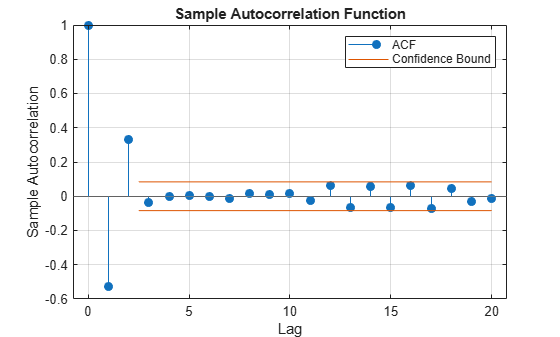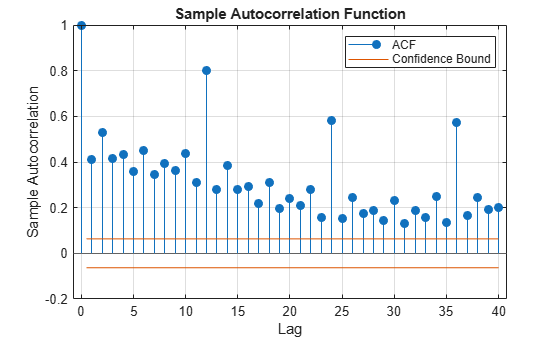# autocorr

Sample autocorrelation

## Syntax

``[acf,lags] = autocorr(y)``
``ACFTbl = autocorr(Tbl)``
``````[___,bounds] = autocorr(___)``````
``[___] = autocorr(___,Name=Value)``
``autocorr(___)``
``autocorr(ax,___)``
``````[___,h] = autocorr(___)``````

## Description

example

````[acf,lags] = autocorr(y)` returns the sample autocorrelation function (ACF) `acf` and associated lags `lags` of the univariate time series `y`.```

example

````ACFTbl = autocorr(Tbl)` returns the table `ACFTbl` containing variables for the sample ACF and associated lags of the last variable in the input table or timetable `Tbl`. To select a different variable in `Tbl`, for which to compute the ACF, use the `DataVariable` name-value argument.```

example

``````[___,bounds] = autocorr(___)``` uses any input-argument combination in the previous syntaxes, and returns the output-argument combination for the corresponding input arguments and the approximate upper and lower confidence bounds `bounds` on the ACF.```

example

````[___] = autocorr(___,Name=Value)` uses additional options specified by one or more name-value arguments. For example, `autocorr(Tbl,DataVariable="RGDP",NumLags=10,NumSTD=1.96)` returns 10 lags of the sample ACF of the table variable `"RGDP"` in `Tbl` and 95% confidence bounds.```

example

````autocorr(___)` plots the sample ACF of the input series with confidence bounds.```
````autocorr(ax,___)` plots on the axes specified by `ax` instead of the current axes (`gca`). `ax` can precede any of the input argument combinations in the previous syntaxes.```
``````[___,h] = autocorr(___)``` plots the sample ACF of the input series and additionally returns handles to plotted graphics objects. Use elements of `h` to modify properties of the plot after you create it.```

## Examples

collapse all

Compute the ACF of a univariate time series. Input the time series data as a numeric vector.

Load the quarterly real GDP series in `Data_GDP.mat`. Plot the series, which is stored in the numeric vector `Data`.

```load Data_GDP plot(Data)```The series exhibits exponential growth.

Compute the returns of the series.

`ret = price2ret(Data);`

`ret` is a series of real GDP returns; it has one less observation than the real GDP series.

Compute the ACF of the real GDP returns, and return the associated lags.

```[acf,lags] = autocorr(ret); [acf lags]```
```ans = 21×2 1.0000 0 0.3329 1.0000 0.1836 2.0000 -0.0216 3.0000 -0.1172 4.0000 -0.1632 5.0000 -0.0870 6.0000 -0.0707 7.0000 -0.0380 8.0000 0.0554 9.0000 ⋮ ```

Let ${\mathit{y}}_{\mathit{t}}$ be the real GDP return at time $\mathit{t}$. In general, `acf(``j``)` = Corr(${\mathit{y}}_{\mathit{t}}$,${\mathit{y}}_{\mathit{t}-\mathrm{lags}\left(\mathit{j}\right)}$). Therefore, `acf(1)` = Corr(${\mathit{y}}_{\mathit{t}}$,${\mathit{y}}_{\mathit{t}}$) = `1.0000`, `acf(2)` = Corr(${\mathit{y}}_{\mathit{t}}$,${\mathit{y}}_{\mathit{t}-1}$) = `0.3329`, and so on.

Compute the ACF of a time series, which is one variable in a table.

Load the electricity spot price data set `Data_ElectricityPrices.mat`, which contains the daily spot prices in the timetable `DataTimeTable`.

```load Data_ElectricityPrices.mat DataTimeTable.Properties.VariableNames```
```ans = 1x1 cell array {'SpotPrice'} ```

Plot the series.

`plot(DataTimeTable.SpotPrice)`The time series plot does not clearly indicate an exponential trend or unit root.

Compute the ACF of the raw spot price series.

`ACFTbl = autocorr(DataTimeTable)`
```ACFTbl=21×2 table Lags ACF ____ _______ 0 1 1 0.55405 2 0.38251 3 0.31713 4 0.25107 5 0.21436 6 0.21275 7 0.19396 8 0.18292 9 0.18826 10 0.19476 11 0.19043 12 0.19963 13 0.19397 14 0.19957 15 0.25495 ⋮ ```

`autocorr` returns the results in the table `ACFTbl`, where variables correspond to the ACF (`ACF`) and associated lags `(Lags)`.

By default, `autocorr` computes the ACF of the last variable in the table. To select a variable from an input table, set the `DataVariable` option.

Consider the electricity spot prices in Compute ACF of Table Variable.

Load the electricity spot price data set `Data_ElectricityPrices.mat`. Compute the ACF and return the ACF confidence bounds.

```load Data_ElectricityPrices [ACFTbl,bounds] = autocorr(DataTimeTable)```
```ACFTbl=21×2 table Lags ACF ____ _______ 0 1 1 0.55405 2 0.38251 3 0.31713 4 0.25107 5 0.21436 6 0.21275 7 0.19396 8 0.18292 9 0.18826 10 0.19476 11 0.19043 12 0.19963 13 0.19397 14 0.19957 15 0.25495 ⋮ ```
```bounds = 2×1 0.0532 -0.0532 ```

Assuming the spot prices follow a Gaussian white noise series, an approximate 95.4% confidence interval on the ACF is (-0.0532, 0.0532).

Although various estimates of the sample autocorrelation function exist, `autocorr` uses the form in Box, Jenkins, and Reinsel, 1994. In their estimate, they scale the correlation at each lag by the sample variance (`var(y,1)`) so that the autocorrelation at lag 0 is unity. However, certain applications require rescaling the normalized ACF by another factor.

Simulate 1000 observations from the standard Gaussian distribution.

```rng(1); % For reproducibility y = randn(1000,1);```

Compute the normalized and unnormalized sample ACF.

```[normalizedACF, lags] = autocorr(y,NumLags=10); unnormalizedACF = normalizedACF*var(y,1);```

Compare the first 10 lags of the sample ACF with and without normalization.

`[lags normalizedACF unnormalizedACF]`
```ans = 11×3 0 1.0000 0.9960 1.0000 -0.0180 -0.0180 2.0000 0.0536 0.0534 3.0000 -0.0206 -0.0205 4.0000 -0.0300 -0.0299 5.0000 -0.0086 -0.0086 6.0000 -0.0108 -0.0107 7.0000 -0.0116 -0.0116 8.0000 0.0309 0.0307 9.0000 0.0341 0.0340 ⋮ ```

Specify the MA(2) model:

`${y}_{t}={\epsilon }_{t}-0.5{\epsilon }_{t-1}+0.4{\epsilon }_{t-2},$`

where ${\epsilon }_{t}$ is Gaussian with mean 0 and variance 1.

```rng(1); % For reproducibility Mdl = arima(MA={-0.5 0.4},Constant=0,Variance=1)```
```Mdl = arima with properties: Description: "ARIMA(0,0,2) Model (Gaussian Distribution)" SeriesName: "Y" Distribution: Name = "Gaussian" P: 0 D: 0 Q: 2 Constant: 0 AR: {} SAR: {} MA: {-0.5 0.4} at lags [1 2] SMA: {} Seasonality: 0 Beta: [1×0] Variance: 1 ```

Simulate 1000 observations from `Mdl`.

`y = simulate(Mdl,1000);`

Plot the ACF of the simulated series. Specify that the series is an MA(2) process.

`autocorr(y,NumMA=2)`The ACF cuts off after the second lag. This behavior is indicative of an MA(2) process.

Specify the multiplicative seasonal ARMA $\left(2,0,1\right)×\left(3,0,0{\right)}_{12}$ model:

`$\left(1-0.75L-0.15{L}^{2}\right)\left(1-0.9{L}^{12}+0.5{L}^{24}-0.5{L}^{36}\right){y}_{t}=2+{\epsilon }_{t}-0.5{\epsilon }_{t-1},$`

where ${\epsilon }_{t}$ is Gaussian with mean 0 and variance 1.

```Mdl = arima(AR={0.75,0.15},SAR={0.9,-0.5,0.5}, ... SARLags=[12 24 36],MA=-0.5,Constant=2, ... Variance=1);```

Simulate data from `Mdl`.

```rng(1); % For reproducibility y = simulate(Mdl,1000); ```

Plot the default autocorrelation function (ACF).

```figure autocorr(y)```The default correlogram does not display the dependence structure for higher lags.

Plot the ACF for 40 lags.

```figure autocorr(y,NumLags=40)```The correlogram shows the larger correlations at lags 12, 24, and 36.

## Input Arguments

collapse all

Observed univariate time series for which `autocorr` computes or plots the ACF, specified as a numeric vector.

Data Types: `double`

Time series data, specified as a table or timetable. Each row of `Tbl` contains contemporaneous observations of all variables.

Specify a single series (variable) by using the `DataVariable` argument. The selected variable must be numeric.

Axes on which to plot, specified as an `Axes` object.

By default, `autocorr` plots to the current axes (`gca`).

Note

Specify missing observations using `NaN`. The `autocorr` function treats missing values as missing completely at random.

### Name-Value Arguments

Specify optional pairs of arguments as `Name1=Value1,...,NameN=ValueN`, where `Name` is the argument name and `Value` is the corresponding value. Name-value arguments must appear after other arguments, but the order of the pairs does not matter.

Before R2021a, use commas to separate each name and value, and enclose `Name` in quotes.

Example: `autocorr(Tbl,DataVariable="RGDP",NumLags=10,NumSTD=3)` plots `10` lags of the sample ACF of the variable `"RGDP"` in `Tbl`, and displays confidence bounds consisting of `3` standard errors away from 0.

Number of lags in the sample ACF, specified as a positive integer. `autocorr` uses lags `0:NumLags` to estimate the ACF.

The default is ```min([20,T – 1])```, where `T` is the effective sample size of the input time series.

Example: `autocorr(y,NumLags=10)` plots the sample ACF of `y` for lags `0` through `10`.

Data Types: `double`

Number of lags in a theoretical MA model of the input time series, specified as a nonnegative integer less than `NumLags`.

`autocorr` uses `NumMA` to estimate confidence bounds.

• For lags > `NumMA`, `autocorr` uses Bartlett’s approximation  to estimate the standard errors under the model assumption.

• If `NumMA` = `0`, then `autocorr` assumes that the input time series is a Gaussian white noise process with a standard error of approximately $1/\sqrt{T},$ where T is the effective sample size of the input time series.

Example: `autocorr(y,NumMA=10)` specifies that `y` is an MA(`10`) process and plots confidence bounds for all lags greater than `10`.

Data Types: `double`

Number of standard errors in the confidence bounds, specified as a nonnegative scalar. For all lags greater than `NumMA`, the confidence bounds are 0 ±`NumSTD*`$\stackrel{^}{\sigma }$, where $\stackrel{^}{\sigma }$ is the estimated standard error of the sample autocorrelation.

The default yields the approximate 95% confidence bounds.

Example: `autocorr(y,NumSTD=1.5)` plots the ACF of `y` with confidence bounds `1.5` standard errors away from 0.

Data Types: `double`

Variable in `Tbl` for which `autocorr` computes the ACF, specified as a string scalar or character vector containing a variable name in `Tbl.Properties.VariableNames`, or an integer or logical vector representing the index of a name. The selected variable must be numeric.

Example: `DataVariable="GDP"`

Example: `DataVariable=[false true false false]` or `DataVariable=2` selects the second table variable.

Data Types: `double` | `logical` | `char` | `string`

## Output Arguments

collapse all

Sample ACF, returned as a numeric vector of length `NumLags` + `1`. `autocorr` returns `acf` only when you supply the input `y`.

The elements of `acf` correspond to lags 0,1,2,..., `NumLags` (that is, elements of `lags`). For all time series, the lag 0 autocorrelation `acf(1)` = `1`.

ACF lags, returned as a numeric vector with elements `0:NumLags`. `autocorr` returns `lags` only when you supply the input `y`.

Sample ACF, returned as a table with variables for the outputs `acf` and `lags`. `autocorr` returns `ACFTbl` when you supply the input `Tbl`.

Approximate upper and lower confidence bounds assuming the input series is an MA(`NumMA`) process, returned as a two-element numeric vector. The `NumSTD` option specifies the number of standard errors in the confidence bounds.

Handles to plotted graphics objects, returned as a graphics array. `h` contains unique plot identifiers, which you can use to query or modify properties of the plot.

collapse all

### Autocorrelation Function

The autocorrelation function measures the correlation between the univariate time series yt and yt + k, where k = 0,...,K and yt is a stochastic process.

According to , the autocorrelation for lag k is

`${r}_{k}=\frac{{c}_{k}}{{c}_{0}},$`

where

• ${c}_{k}=\frac{1}{T}\sum _{t=1}^{T-k}\left({y}_{t}-\overline{y}\right)\left({y}_{t+k}-\overline{y}\right).$

• c0 is the sample variance of the time series.

Suppose that q is the lag beyond which the theoretical ACF is effectively 0. Then, the estimated standard error of the autocorrelation at lag k > q is

`$SE\left({r}_{k}\right)=\sqrt{\frac{1}{T}\left(1+2\sum _{j=1}^{q}{r}_{j}^{2}\right)}.$`

If the series is completely random, then the standard error reduces to $1/\sqrt{T}$.

### Missing Completely at Random

Observations of a random variable are missing completely at random if the tendency of an observation to be missing is independent of both the random variable and the tendency of all other observations to be missing.

## Tips

• To plot the ACF without confidence bounds, set `NumSTD=0`.

## Algorithms

• If the input series is a fully observed series (that is, it does not contain any `NaN` values), `autocorr` uses a Fourier transform to compute the ACF in the frequency domain, then converts back to the time domain using an inverse Fourier transform.

• If the input series is not fully observed (that is, it contains at least one `NaN` value), `autocorr` computes the ACF at lag k in the time domain, and includes in the sample average only those terms for which the cross product ytyt+k exists. Consequently, the effective sample size is a random variable.

• `autocorr` plots the ACF when you do not return any output or when you return the fourth output `h`.

 Box, George E. P., Gwilym M. Jenkins, and Gregory C. Reinsel. Time Series Analysis: Forecasting and Control. 3rd ed. Englewood Cliffs, NJ: Prentice Hall, 1994.

 Hamilton, James D. Time Series Analysis. Princeton, NJ: Princeton University Press, 1994.